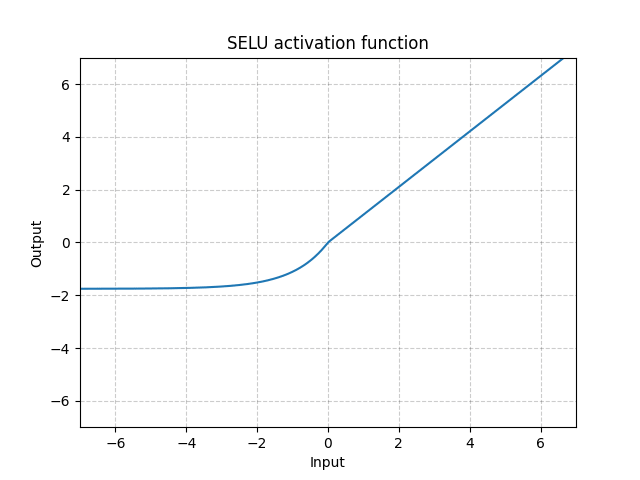/PyTorch

# SELU

class torch.nn.SELU(inplace: bool = False) [source]

Applied element-wise, as:

$\text{SELU}(x) = \text{scale} * (\max(0,x) + \min(0, \alpha * (\exp(x) - 1)))$

with $\alpha = 1.6732632423543772848170429916717$ and $\text{scale} = 1.0507009873554804934193349852946$ .

More details can be found in the paper Self-Normalizing Neural Networks .

Parameters

inplace (bool, optional) – can optionally do the operation in-place. Default: False

Shape:
• Input: $(N, *)$ where * means, any number of additional dimensions
• Output: $(N, *)$ , same shape as the inputExamples:

>>> m = nn.SELU()
>>> input = torch.randn(2)
>>> output = m(input)


© 2019 Torch Contributors
Licensed under the 3-clause BSD License.
https://pytorch.org/docs/1.7.0/generated/torch.nn.SELU.html